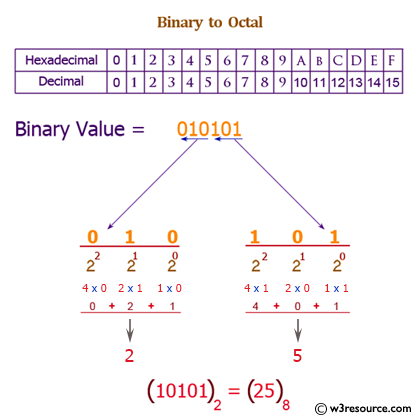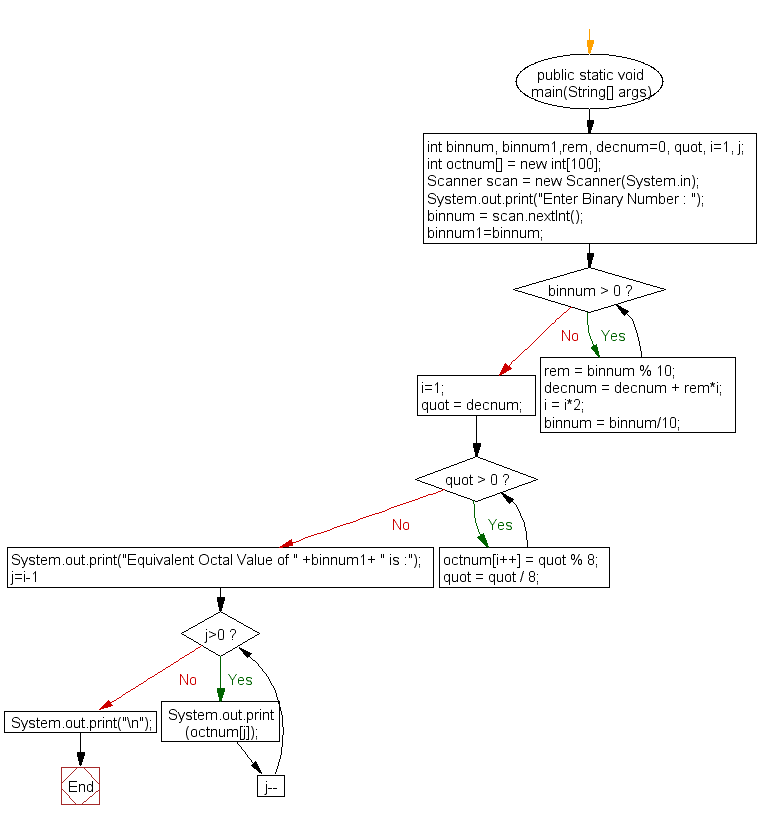﻿ Java exercises: Convert a binary number to a Octal number - w3resource# Java Exercises: Convert a binary number to a Octal number

## Java Basic: Exercise-24 with Solution

Write a Java program to convert a binary number to a Octal number.

Binary number: A binary number is a number expressed in the base-2 numeral system or binary numeral system. This system uses only two symbols: typically 0(zero) and 1(one).

Octal number: The octal numeral system is the base-8 number system, and uses the digits 0 to 7.

Test Data:
Input a binary number: 111

Pictorial Presentation: Binary to Octal numberSample Solution:

Java Code:

``````import java.util.*;
public class Exercise24 {
public static void main(String[] args)
{
int binnum, binnum1,rem, decnum=0, quot, i=1, j;
int octnum[] = new int;
Scanner scan = new Scanner(System.in);
System.out.print("Input a Binary Number : ");
binnum = scan.nextInt();
binnum1=binnum;

while(binnum > 0)
{
rem = binnum % 10;
decnum = decnum + rem*i;
//System.out.println(rem);
i = i*2;
binnum = binnum/10;
}

i=1;
quot = decnum;

while(quot > 0)
{
octnum[i++] = quot % 8;
quot = quot / 8;
}

System.out.print("Equivalent Octal Value of " +binnum1+ " is :");
for(j=i-1; j>0; j--)
{
System.out.print(octnum[j]);
}
System.out.print("\n");

}
}
```
```

Sample Output:

```Enter Binary Number : 111
Equivalent Octal Value of 111 is :7
```

Flowchart:Java Code Editor:

What is the difficulty level of this exercise?

Test your Programming skills with w3resource's quiz.

﻿

## Java: Tips of the Day

getEnumMap

Converts to enum to Map where key is the name and value is Enum itself.

```public static <E extends Enum<E>> Map<String, E> getEnumMap(final Class<E> enumClass) {
return Arrays.stream(enumClass.getEnumConstants())
.collect(Collectors.toMap(Enum::name, Function.identity()));
}
```

Ref: https://bit.ly/3xXcFZt